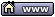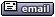flat assembler
Message board for the users of flat assembler.flat assembler > Windows > FPU fxtract i am confused.
Author
 ThreadRoman

Joined: 21 Apr 2012
Posts: 497
Code:
```fltcc      dd 1.3545
fld     dword [fltcc]
fxtract
fistp  dword [Status+200] ;i get 1.0 mantise
fistp  dword [Status+240] ;i get 0 exponent
```

I expect 1 and 3545
I want convert float to text.19 Jul 2019, 08:49revolution
When all else fails, read the source

Joined: 24 Aug 2004
Posts: 16737
Location: In your JS exploiting you and your system
1.3545 == 1.3545 * 2^0

So the exponent is zero
And the mantissa is 1.3545

If you want the fraction then fxtract won't do that. You might want to truncate, or round, the value and then get the fraction by subtracting.19 Jul 2019, 09:31Roman

Joined: 21 Apr 2012
Posts: 497
I try do round, the value and then get the fraction by subtracting.
Its work on 1.3545 but not good on big float.

I try on 2255752.3545 and get 0.25 when do round and subtracting.

I thought fxtract more accurate.19 Jul 2019, 09:44revolution
When all else fails, read the source

Joined: 24 Aug 2004
Posts: 16737
Location: In your JS exploiting you and your system
You are using dword floats so you will lose precision. Try with qword floats for numbers with many digits before the fraction.19 Jul 2019, 11:34fpissarra

Joined: 10 Apr 2019
Posts: 58
For the float type, 32 bits long, the binary structure is (from msb to lsb):

seeeeeeeefffffffffffffffffffffff

Where s, e (8 bits) and f (23 bits) are integers for the signal (1 means negative value), expoent and "significant", respectively. The floating point value is, for normalized floats, given by:

v = (-1)^s * (1 + f/(2^23}) * 2^(e-127)

fxtract will put in ST(0) the expoent (e-127) and, on top of the stack, (1 + f/(2^23)). Notice the significant is stored in floating point as well.24 Jul 2019, 12:13fpissarra

Joined: 10 Apr 2019
Posts: 58
Here what I think you want:
Code:
```x:  dd 1.3545
integerPart: dd ?
fractionalPart: dd ?
...
sub esp,4
fld dword [x]       ; push x
fist dword [esp]    ; stores integral part of x.
fild dword [esp]    ; pushes integral part of x

; now, st(0) = int(x), st(1) = x.

fst dword [integerPart]
fsubp st(1),st(0)           ; st(1) = st(1) - st(0), pops (st(1) becomes st(0)).
fstp dword [fracionalPart]  ; store st(0) and pops (clears stack).
...
```

Notice: Both integralPart and fractionalPart are floating point (float) types.

Change fst dword [integerPart] to fist dword [integerPart] if you want to store the integer part as 'integer'.

Assuming, of course, x is a valid floating point value (not NAN or INF).

Beware of the binary precision of the type as well... float has only 24 bits of precision (values greater than 2^24-1 cannot be represented accurately - will loose lower bits) - if you store the integer part in 'integer' this will be an issue.24 Jul 2019, 14:10fpissarra

Joined: 10 Apr 2019
Posts: 58
Notice that with SSE the routine fragment is easier:
Code:
```x: dd 1.3545
integerPart: dd ?
fractionalPart: dd ?
...
movss xmm0,dword [x]

cvttss2si eax,xmm0
cvtsi2ss xmm1,eax  ; xmm1 = int(x).

subss xmm0,xmm1  ; strips the integer part from xmm0.

movss dword [integerPart],xmm1
movss dword [fractionalPart],xmm0
...    ```

Again, both integerPart and fractionalPart are floats.

But the above routine suffers from the same problem of precision as before... converting xmm0 to eax can loose lower bits and very big values cannot be converted (what if xmm0 == 1.3275*10^30?).

We could convert float to double (cvtss2sd), use an integral part greater than 32 bits and convert double to integer (cvtsd2si) with 64 bits operand, but this only would pospone the problem... BTW, f87 fsti and fldi instructions have the same problem...24 Jul 2019, 14:21Roman

Joined: 21 Apr 2012
Posts: 497
This code have problem if x = 2.94 !
Code:
```movss xmm0,dword [x]

cvttss2si eax,xmm0 ;eax = 3.0
cvtsi2ss xmm1,eax  ; xmm1 = int(x).

subss xmm0,xmm1  ; strips the integer part from xmm0.    ```

Best way use roundss.24 Jul 2019, 15:59fpissarra

Joined: 10 Apr 2019
Posts: 58
Hummmm... I don't know... the exact same code, but writen in C, works perfectly:
Code:
```/* func.c */
void f( float x, float *i, float *f )
{
int t = x;
*i = t;
*f = x - t;
}    ```

Which, with GCC (intel syntax), gives:
Code:
```\$ objdump -dM intel func.o
func.o:     file format elf64-x86-64

Disassembly of section .text:

0000000000000000 <f>:
0: f3 0f 2c c0           cvttss2si eax,xmm0
4: 66 0f ef c9           pxor   xmm1,xmm1
8: f3 0f 2a c8           cvtsi2ss xmm1,eax
c: f3 0f 5c c1           subss  xmm0,xmm1
10: f3 0f 11 0f           movss  DWORD PTR [rdi],xmm1
14: f3 0f 11 06           movss  DWORD PTR [rsi],xmm0
18: c3                    ret    ```

This pxor isn't necessary, but even adding it to the SAME routine, writen in ASM, for that value 2.94, gives 3 and -0.05999?! Why!?

Here's the 'test.c' test:
Code:
```/* test.c */
#include <stdio.h>

/* Defined in func.c */
void f( float, float *, float * );

int main( void )
{
float x = 2.94;
float i, fr;

f( x, &i, &fr );
printf( "%f -> (int) %f, (frac) %f\n", x, i, fr );
}    ```24 Jul 2019, 18:44fpissarra

Joined: 10 Apr 2019
Posts: 58
AHHHHHHHHHHHHHHHHHHHH... Because IT ISN'T the same routine... Notice the cvttss2si instruction used by GCC. This is truncation (not using rounding!)... aahhhhhhhh!I was testing with cvtss2si before... cvttss2si will get the integer part correctly.24 Jul 2019, 18:52Roman

Joined: 21 Apr 2012
Posts: 497
Thanks for cvttss2si.
I not using cvttss2si.

Last edited by Roman on 25 Jul 2019, 11:16; edited 1 time in total24 Jul 2019, 18:58fpissarra

Joined: 10 Apr 2019
Posts: 58
A good explanation for floating point encoding I've read is this: Notice the equation which give you the actual value is (as I showed earlier):

v = (-1)^s * (1 + f/(2^23)) * 2^E

Where E is e-127. Here E is 8 bits long and f is an integer 23 bits long. Consider if E=0 and f=0, the value is 1 (let's consider s=0 for the entire text). If f is maximum (all 23 bits set) the value is closer to 2 (f can go to (2^23)-1, maximum). It means, what you are doing changing the value of E is marking the begining of a range between 2^E (f=0) and 2^(E+1) (for f=max), excluding the last value.

So... to get E you can:

E=floor(log2(v))

For example... consider 0.135: E=floor(log2(0.135))=-3. This gives you the range between 2^-3 to 2^-2 (0.125 to 0.25).

Now.... 0.135 is 0.01 plus 0.125 (the start of the range). What is the proportion of 0.01 in the range between 2^-3 and 2^-2? p=(0.01/(2^-2 - 2^-3))=0.08.

But if f is 23 bits long, 0.08*(2^23)=671089 (f = ceil(p*2^23)).

We got: f = 671089 = 0b00010100011110101110001 (23 bits);
E = -3 (E=e-127 -> e=E+127=124 = 0b01111100 (8 bits);
and s = 0

So: v = 0b0_01111100_00010100011110101110001 = 0x3E0A3D71

If you print a float in hex, as an integer:
Code:
```#include <stdio.h>

int main( void )
{
float f = 0.135;
int i = *(int *)&f;

printf( "%#x\n", i );
}    ```

You'll get exactly that final value of 0x3e0a3d71.

For 0.135, If you use fextract, you'll get ( approx 1+0.08 ) as "significant" and -3 as expoent, as 1.08*2^-3 = 0.13524 Jul 2019, 22:54fpissarra

Joined: 10 Apr 2019
Posts: 58
As you have notice (probably), floating point values aren't a representation of REAL numbers, but of RATIONAL numbers. It stores a ratio (f/(2^23))*2^E. The error between two values is 2^-23 multiplied by the scale 2^E.

Consider a big number: v=24734834783, for instance... E=floor(log2(v))=34. The error between to closer values is 2^-23*2^34 = 2^(34 - 23) = 2^11... Or, 2048. Since the range starts at 2^34 (17179869184), the next possible value is 17179871232, the next is +2048 away, and so on...

There is no way you can represent exactly 17179869185 (start of the range +1)! In fact, 24734834783 cannot be represented exactly as well, because it is between valid values (between 24734834688 and 24734836736, in this scale)...

Just to satisfy the curiosity: the value obtained will be 24734834688 (rounding to nearest), and the significant part is approx 1.43976 with expoent 34.24 Jul 2019, 23:14AsmGuru62

Joined: 28 Jan 2004
Posts: 1394
To convert ST0 to text -- FBSTP is most likely the way to do it.
http://www.website.masmforum.com/tutorials/fptute/fpuchap6.htm#fbstp26 Jul 2019, 21:52fpissarra

Joined: 10 Apr 2019
Posts: 58
AsmGuru62 wrote:
To convert ST0 to text -- FBSTP is most likely the way to do it.
http://www.website.masmforum.com/tutorials/fptute/fpuchap6.htm#fbstp

It works for INTEGER values stored in ST(0) - otherwise, the value will be rounded. And notice the value can be big enough NOT to fit in the 18 byte array (36 digits), since fbstp stores the 'packed bcd'.

Anyway... I didn't recall for fbstp, nice tip!26 Jul 2019, 23:13AsmGuru62

Joined: 28 Jan 2004
Posts: 1394
Yes, I forgot to add that you need to multiply ST0 by 10 in magnitude of # of digits, like you need 6 digits after the decimal then by 1000000.26 Jul 2019, 23:33Display posts from previous: All Posts1 Day7 Days2 Weeks1 Month3 Months6 Months1 Year Oldest FirstNewest FirstJump to: Select a forum Official----------------AssemblyPeripheria General----------------MainDOSWindowsLinuxUnixMenuetOS Specific----------------MacroinstructionsCompiler InternalsIDE DevelopmentOS ConstructionNon-x86 architecturesHigh Level LanguagesProgramming Language DesignProjects and IdeasExamples and Tutorials Other----------------FeedbackHeapTest Area

Forum Rules:
 You cannot post new topics in this forumYou cannot reply to topics in this forumYou cannot edit your posts in this forumYou cannot delete your posts in this forumYou cannot vote in polls in this forumYou cannot attach files in this forumYou can download files in this forum